# Find the regular expression for the given Finite automata with state elimination method

## Problem

Generate RE for a given finite automata by using the state elimination method.

## Solution

There are two methods for converting a Deterministic Finite Automata (DFA) to Regular expression (RE).

• Arden's Theorem Method.
• State Elimination Method.

The given automata is as follows−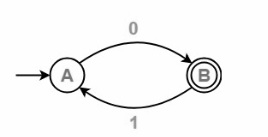## Step 1

Initial state is A that has an incoming edge.

So, we have to create a new initial state qi as follows−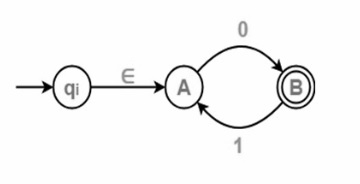## Step 2

Initial state is B that has an incoming edge.

So, we have to create a new final state qf as given below−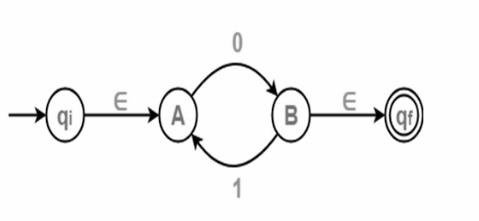## Step 3

Now, try to eliminate all intermediate states one by one.

First eliminate state A

There is a path from state qi to B via A.

So, after eliminating state A, we can connect a direct path from qi to B having cost.

ε.0=0

There is a loop on state B using State A.

So, after eliminating state A, we can draw a direct loop on state B having cost.

1.0=10

After eliminating A, we get the following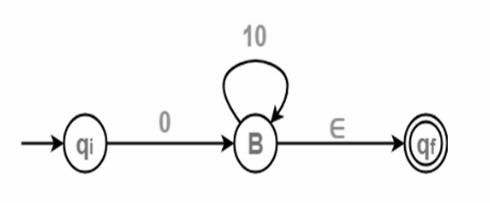## Step 4

Now eliminate State B

There is a path from state qi to qf via state B.

So, after eliminating B, we can draw a direct path from qi to state qf having cost

0.(10)* ε=0(10)*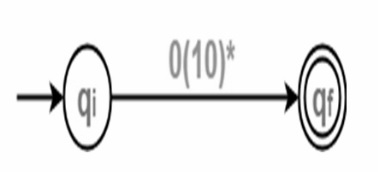Final Regular Expression is = 0(10)*